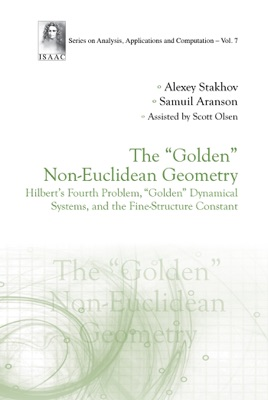• 429,00 kr

## Utgivarens beskrivning

This unique book overturns our ideas about non-Euclidean geometry and the fine-structure constant, and attempts to solve long-standing mathematical problems. It describes a general theory of "recursive" hyperbolic functions based on the "Mathematics of Harmony," and the "golden," "silver," and other "metallic" proportions. Then, these theories are used to derive an original solution to Hilbert's Fourth Problem for hyperbolic and spherical geometries. On this journey, the book describes the "golden" qualitative theory of dynamical systems based on "metallic" proportions. Finally, it presents a solution to a Millennium Problem by developing the Fibonacci special theory of relativity as an original physical-mathematical solution for the fine-structure constant. It is intended for a wide audience who are interested in the history of mathematics, non-Euclidean geometry, Hilbert's mathematical problems, dynamical systems, and Millennium Problems.
Contents:The Golden Ratio, Fibonacci Numbers, and the "Golden" Hyperbolic Fibonacci and Lucas FunctionsThe Mathematics of Harmony and General Theory of Recursive Hyperbolic FunctionsHyperbolic and Spherical Solutions of Hilbert's Fourth Problem: The Way to the Recursive Non-Euclidean GeometriesIntroduction to the "Golden" Qualitative Theory of Dynamical Systems Based on the Mathematics of HarmonyThe Basic Stages of the Mathematical Solution to the Fine-Structure Constant Problem as a Physical Millennium ProblemAppendix: From the "Golden" Geometry to the Multiverse
Readership: Advanced undergraduate and graduate students in mathematics and theoretical physics, mathematicians and scientists of different specializations interested in history of mathematics and new mathematical ideas.